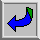## Zeno’s Paradoxes: Some Mathematical Preliminaries

The sequence:

1/2, 1/4, 1/8, 1/16, . . . .

has infinitely many terms, each of which is of finite size greater than zero. The sequence has no last term, but it does have a limit, namely zero. (Roughly: no matter how close to zero you want to get, there is a term in the sequence that gets you at least that close.)

If we add together all the terms in the sequence above, we get an infinite series:

1/2 + 1/4 + 1/8 + 1/16 + . . . .

To calculate the sum of this series, one must consider the infinite sequence <S1, S2, S3, . . . .>, where:

S1 = 1/2

S2 = 1/2 + 1/4

S3 = 1/2 + 1/4 + 1/8

etc.

<S1, S2, S3, . . . .> is called the sequence of partial sums of the infinite series 1/2 + 1/4 + 1/8 + . . . .

Our sequence of partial sums is thus:

1/2, 3/4, 7/8, 15/16, . . . .

This sequence also has a limit, namely 1.
 The sum of an infinite series is defined as the limit (where one exists) of the sequence of partial sums.

Therefore, our infinite series has a finite sum. That is:

1/2 + 1/4 + 1/8 + . . . . = 1.

Zeno seems, in some sense, to recognize that this is so, since he concedes that a race-course of length d can be divided into infinitely many parts, 1/2d + 1/4d + 1/8d + . . . . That is, he concedes that

d/2 + d/4 + d/8 + . . . = d.

But there is evidence that he also thought that anything divisible into infinitely many parts must be infinitely large. (Cf. the second limb of the argument against plurality [as recorded by Simplicius, in DK1].) Hence he might have felt entitled to the conclusion that to traverse a finite race-course one must travel an infinitely great distance.

Is Zeno simply confused? Not necessarily. For he may think that this bizarre conclusion is one that his opponent (the pluralist), but not he, must accept. If, as Zeno thinks, the very notion of plurality is incoherent, it would not be surprising for him to think that a plurality (if there were one) would have to be both finite and infinite.Return to lecture on Zeno against pluralityGo to next lecture on Zeno’s race course, part 2Return to the PHIL 320 Home Page